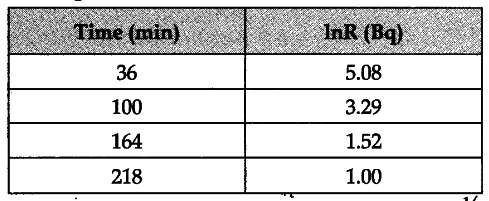# The following table shows some measurements of the decay rate of a radio nuclei sample

The following table shows some measurements of the decay rate of a radio nuclei sample. Find the disintegration constant.$R=R_{ 0 }{ e }^{ -\lambda t }$
$In\ R=In\ {{R}{0}}-\lambda t$
$In\ R=-\lambda t+In\ {{R} {0}}$
Slop of in R V/s t is$’-\lambda '$
\begin{align} & -\lambda =\frac{0-1.52}{218-164} \ & \lambda =0.028\ \min it{{e}^{-1}} \ \end{align}